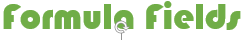## Formula fieldsWhat is a formula field? It is a read only field, the value of formulafiled evaluate from expression defined by us. If we update any value in the expression, it automatically updates formula field value. We can create formula fields in both standard and custom object.

We can return formule result in 7 ways those are:

1. Checkbox: Calculate a boolean value
2. Currency: Calculate a dollar or other currency amount and automatically format the field as a currency amount.
3. Date: Calculate a date, for example, by adding or subtracting days to other dates.
4. Date/Time: Calculate a date/time, for example, by adding a number of hours or days to another date/time.
5. Number: Calculate a numeric value.
6. Percent: Calculate a percent and automatically add the percent sign to the number.
7. Text: Create a text string, for example, by concatenating other text fields.

Creating this type of filed is like creating a normal salesforce field with data type formulae and we need to select result typed & provide expression.

Find more on salesforce formulafields

For Examples see salesforce reference